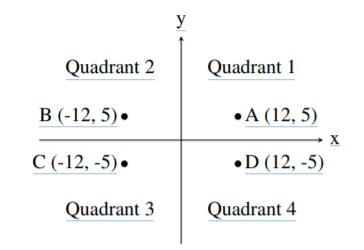TORONTO KIDS COMPUTER CLUB | Aurora Saturday 14:30 Python Practice 20.06.13.
17719

# Aurora Saturday 14:30 Python Practice 20.06.13.

## 18 Jun Aurora Saturday 14:30 Python Practice 20.06.13.

Question 1:
A common problem in mathematics is to determine which quadrant a given point lies in. There are four quadrants, numbered from 1 to 4, as shown in the diagram below:For example, the point A, which is at coordinates (12, 5) lies in quadrant 1 since both its x and y values are positive, and point B lies in quadrant 2 since its x value is negative and its y value is positive.

Your job is to take a point and determine the quadrant it is in. You can assume that neither of the two coordinates will be 0.

Input Specification
The first line of input contains the integer x (−1000 ≤ x ≤ 1000; x 6= 0). The second line of input contains the integer y (−1000 ≤ y ≤ 1000; y 6= 0).

Output Specification
Output the quadrant number (1, 2, 3 or 4) for the point (x, y).

`Sample Input 1125Output for Sample Input 11Sample Input 29-13Output for Sample Input 24`

Question 2:
Your new cellphone plan charges you for every character you send from your phone. Since you tend to send sequences of symbols in your messages, you have come up with the following compression technique: for each symbol, write down the number of times it appears consecutively, followed by the symbol itself. This compression technique is called run-length encoding.

More formally, a block is a substring of identical symbols that is as long as possible. A block will be represented in compressed form as the length of the block followed by the symbol in that block. The encoding of a string is the representation of each block in the string in the order in which they
appear in the string.

Given a sequence of characters, write a program to encode them in this format.

Input Specification
The first line of input contains the number N, which is the number of lines that follow. The next
N lines will contain at least one and at most 80 characters, none of which are spaces.

Output Specification
Output will be N lines. Line i of the output will be the encoding of the line i + 1 of the input. The encoding of a line will be a sequence of pairs, separated by a space, where each pair is an integer (representing the number of times the character appears consecutively) followed by a space, followed by the character.

Sample Input
4
+++===!!!!
777777……TTTTTTTTTTTT
(AABBC)
3.1415555

Output for Sample Input
3 + 3 = 4 !
6 7 6 . 12 T
1 ( 2 A 2 B 1 C 1 )
1 3 1 . 1 1 1 4 1 1 4 5

Explanation of Output for Sample Input
To see how the first message (on the second line of input) is encoded, notice that there are 3 + symbols, followed by 3 = symbols, followed by 4 ! symbols.